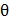# A conducting circular loop made of a thill wire, has area 3.510−3 m2 and resistance 10Ω.  It is placed perpendicular to a time dependent magnetic field B(1) = (0.4T)sin(50t).  The field is uniform in space.  Then the net charge flowing through the loop during t = 0 s and  t = 10 ms is close to: a. 14mC b. 21mC c. 6mC d. 7mC

## Question ID - 50046 :- A conducting circular loop made of a thill wire, has area 3.510−3 m2 and resistance 10Ω.  It is placed perpendicular to a time dependent magnetic field B(1) = (0.4T)sin(50t).  The field is uniform in space.  Then the net charge flowing through the loop during t = 0 s and  t = 10 ms is close to: a. 14mC b. 21mC c. 6mC d. 7mC

3537

Q==A(Bf−Bf)=3.510−3=(3.510−3)(0.4−0)

=1.410−4 = 0.14mC

Next Question :

Two masses m andare connected at the two ends of a massless rigid rod of length ll.  The rod is suspended by a thin wire of torsional constant k at the centre of mass of the rod-mass system (sec figure). Because of torsional constant k, the restoring torque is=kfor angular displacement 0.  If the rod is rotated by0 and released, the tension in it when it passes through its mean position will be:a.b.c.d.